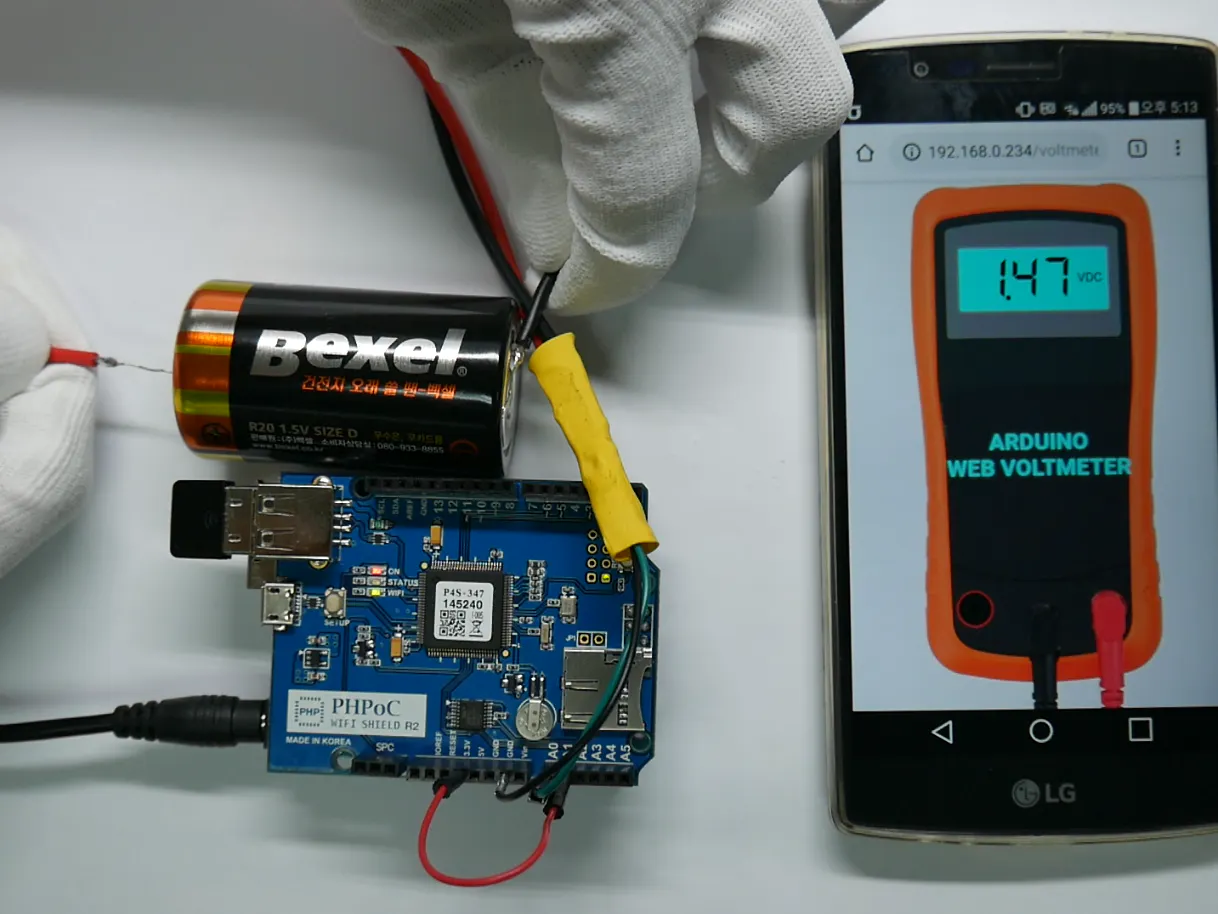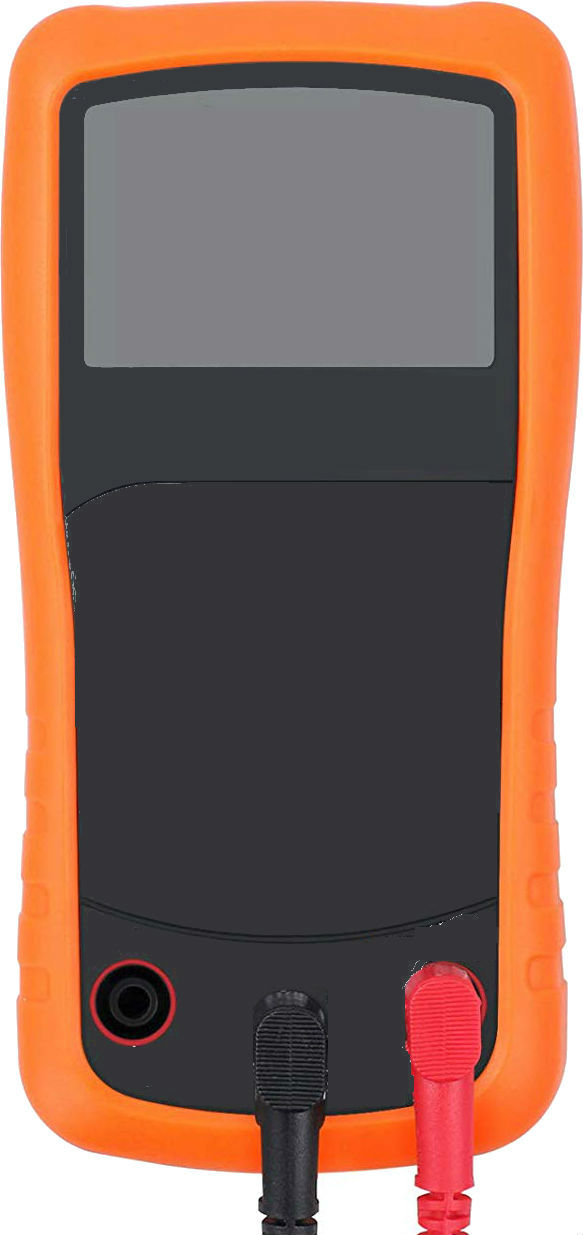Project tutorial# DIY Voltmeter Using Arduino and Smartphone © GPL3+

Voltmeter can measure voltage up to 50VDC. It automatically calibrates when voltage of power supply is unstable.

• 2,792 views
• 30 respects

## Components and supplies

### Demonstration

If you are new to Arduino, you can get started with Arduino Tutorials for newbie.

### How it works

We can use Arduino analog input pin to measure voltage. However, the maximum measurable voltage is 5V.

To increase the measurable voltage, we need to use a voltage divisor

InTheory

We has:

V_measure = (R1 + R2) / R2 * V_in

ratio = (R1 + R2) / R2

=> V_measure = ratio * V_in

If we choose R1 = 10 * R2, we have:

V_measure = 11 * V_in

Since the maximum of the allowable V_in is 5V => The maximum of the measurable voltage is 55V

To limit the current run though Arduino's pin, which may cause damage to Arduino, we need to choose resistor value as big as possible.

I choose R1 = 1 Mohm, R2 = 100Kohm

In Practice

There is some problems in practice

• Value of R1 and R2 has errors. The errors cause the error of V_measure
• Power source of of Arduino may be unstable. It makes the V_REF of analog input is unstable. Therefore, it causes the error in calculation of V_in. => it causes error in calculation of V_measure

These problems are solved on the Calibration part

### Calibration

1. Measure the real value of (R1 + R2) / R2

This need to be done only one time to get the ratio

Wiring as bellow image:

ratio = (R1 + R2) / R2 = V_measure / V_in = A1_read_value / A0_read_value

Code for this calibration => see ResisterCalibration.ino in code part

2. Instability of power supply

Instability of power supply causes instability in voltage reference of analog pin. It causes the wrong measure in voltage calculation.

To solve it, we need to measure the voltage reference frequently.

How to measure the voltage reference?

Connect 3.3V to pin A1

The voltage reference is measured indirectly based on value in A1:

V_reference= 1023 * 3.3 / A1_value

How to calculate the V_measure:

V_in = mapFloat(A0_value, 0, 1023, 0, V_reference);V_measure = ratio * V_in;

Main wiring:

### How to

You can view the voltage on smart phone as follows:

Wiring Tips:

## Code

##### ResistorCalibration.inoArduino
- Wiring as described in Calibration part
- Run this code once time
- Open Serial Plotter
- Copy the ratio value
- Replace this value in line 5 of Voltmeter.ino
```double ratio;

{
for(int i = 0; i < read_time; i++) {
delay(2);
}

// Get average

}

double resistorCalibration()
{
double _ratio;

return _ratio;
}

void setup() {
// initialize serial communications at 9600 bps:
Serial.begin(9600);
}

void loop() {
ratio = resistorCalibration();

Serial.print("ratio:");
Serial.println(ratio);
delay(200);
}
```
##### Voltmeter.inoArduino
This is main code. Before uploading this code, we need to :
- open Serial Monitor
- copy the ratio value and replace value in line 5 of this code
```double V_measure;
double V_in;
double V_reference;

double ratio = 11.59;

double mapFloat(double x, double in_min, double in_max, double out_min, double out_max) {
return (x - in_min) * (out_max - out_min) / (in_max - in_min) + out_min;
}

{

for(int i = 0; i < read_time; i++) {
delay(2);
}

// get average

}

double getVoltageReference()
{
double A1_value = analogReadAverage(A1, 20); // A1 is connected to 3.3v
double V_REF = 1023 * 3.3 / A1_value;

return V_REF;
}

void setup() {
Serial.begin(9600);
}

void loop() {
// update voltage reference
V_reference = getVoltageReference();

// map it to the range of the analog out:
V_in = mapFloat(A0_value, 0, 1023, 0, V_reference);
V_measure = ratio * V_in;

// print the results to the Serial Monitor:
Serial.println(V_measure);

delay(200);
}
```
##### voltmeter.phpPHP
This file is Web User Interface. It need to be uploaded to PHPoC Shield along with background image voltmeter_body.jpg
```<!DOCTYPE html>
<html>
<title>PHPoC Shield - VOLTMETER</title>
<meta name="viewport" content="width=device-width, initial-scale=0.7">
<style>
html {height: 100%;}
body {width: 100%; height: 100%;; text-align: center;}
.container {width: 100%; min-height: 99%; max-height: 100%;}
canvas {
background: url(voltmeter_body.jpg);
background-size:contain;
font-family: 'Segment7Standard';
font-weight: bold;
font-style: italic;
}

</style>
<script>
var COLOR_LCD_TEXT		= "#000000";
var COLOR_LCD_BGR		= "#00FFFF";
var IMG_WIDTH = 583;
var IMG_HEIGHT = 1235;
var METER_WIDTH, METER_HEIGHT;

var ws;
var canvas;
var ctx;
var buffer = "";
var voltage = 0;

function init()
{
canvas = document.getElementById("graph");
ctx = canvas.getContext("2d");
canvas_resize();
update_view(voltage);

var ws_host_addr = "<?php echo _SERVER("HTTP_HOST")?>";

if((navigator.platform.indexOf("Win") != -1) && (ws_host_addr.charAt(0) == "["))
{
// network resource identifier to UNC path name conversion
}

ws = new WebSocket("ws://" + ws_host_addr + "/serial_monitor", "uint8.phpoc");
ws.onopen = ws_onopen;
ws.onclose = ws_onclose;
ws.onmessage = ws_onmessage;
ws.binaryType = "arraybuffer";
}

function ws_onopen()
{

}
function ws_onclose()
{
ws.onopen = null;
ws.onclose = null;
ws.onmessage = null;
ws = null;
}
function ws_onmessage(e_msg)
{
e_msg = e_msg || window.event; // MessageEvent

var u8view = new Uint8Array(e_msg.data);
buffer += String.fromCharCode.apply(null, u8view);
buffer = buffer.replace(/\r\n/g, "\n");
buffer = buffer.replace(/\r/g, "\n");

while(buffer.indexOf("\n") == 0)
buffer = buffer.substr(1);

if(buffer.indexOf("\n") <= 0)
return;

var pos = buffer.lastIndexOf("\n");
var str = buffer.substr(0, pos);
var arr = str.split("\n");
buffer = buffer.substr(pos + 1);

if(arr.length)
{
voltage = parseFloat(arr[arr.length - 1]);
update_view(voltage);
}
}

function update_view(voltage)
{
ctx.clearRect(0, 0, METER_WIDTH, METER_HEIGHT);

var top = METER_HEIGHT * 0.125; // 12.5%
var left = METER_WIDTH * 0.2; // 2%
var width = METER_WIDTH * 0.6; // 6%
var height = METER_HEIGHT * 0.12; // 12%

ctx.fillStyle = COLOR_LCD_BGR;
ctx.rect(left, top, width, height);
ctx.fill();

ctx.font = "italic 75px Segment7Standard";
ctx.textBaseline = "middle";
ctx.textAlign = "end";
ctx.fillStyle = COLOR_LCD_TEXT;
ctx.fillText(voltage.toFixed(2), left + width - 50, top + height / 1.8);

ctx.font = "18px Arial";
ctx.textBaseline = "middle";
ctx.textAlign = "center";
ctx.fillText("VDC", left + width - 25, top + height / 2);

ctx.font = "bold 32px Arial";
ctx.fillStyle = "#00FFFF";
ctx.fillText("ARDUINO", METER_WIDTH / 2, METER_HEIGHT * 0.5);
ctx.fillText("WEB VOLTMETER", METER_WIDTH / 2, METER_HEIGHT * 0.55);
}

function canvas_resize()
{
canvas.width = 0; // to avoid wrong screen size
canvas.height = 0;

var container = document.getElementById("container");

var height = container.clientHeight;
var ratio = height / IMG_HEIGHT;
var width = ratio * IMG_WIDTH;

METER_WIDTH = width;
METER_HEIGHT = height;

canvas.width = METER_WIDTH;
canvas.height = METER_HEIGHT;
update_view(voltage);
}

</script>
<body onresize="canvas_resize()">
<div class="container" id="container">
<canvas id="graph"></canvas>
</div>
</body>
</html>
```

## Schematics

It need to be uploaded to PHPoC Shield#### DIY Voltmeter with Arduino and a Nokia 5110 Display

Project tutorial by Nick Koumaris

• 12,259 views
• 22 respects

• 4,625 views
• 1 comment
• 30 respects

#### DIY Universal CNC Machine

Project tutorial by Arduino “having11” Guy

• 24,775 views
• 83 respects

• 9,850 views
• 14 respects

#### Cheap DIY LASER ALARM - Multi Functional!

Project tutorial by Danny van den Brande

• 5,951 views